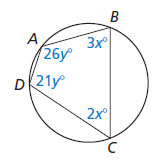Sam G.

# Chapter 10: Inscribed Angles and Polygons

Find the measures of the interior angles of the polygon.angle DAB = ___ degrees

angle ABC = ___ degrees

angle BCD = ___ degrees